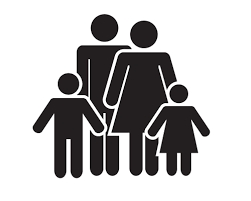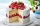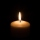# Carla

Carla is 5 years old and Jim is 13 years younger than Peter. One year ago, Peter’s age was twice the sum of Carla’s and Jim’s age. Find the present age of each one of them.

Correct result:

C =  5
J =  6
P =  19

#### Solution:

C=5
J = P-13
P-1 = 2((C-1)+(J-1))

C=5
J = P-13
P-1 = 2•((C-1)+(J-1))

C = 5
J-P = -13
2C+2J-P = 3

C = 5
J = 6
P = 19

Calculated by our linear equations calculator.Our examples were largely sent or created by pupils and students themselves. Therefore, we would be pleased if you could send us any errors you found, spelling mistakes, or rephasing the example. Thank you!

Please write to us with your comment on the math problem or ask something. Thank you for helping each other - students, teachers, parents, and problem authors.Tips to related online calculators
Do you have a linear equation or system of equations and looking for its solution? Or do you have quadratic equation?
Do you want to convert time units like minutes to seconds?

## Next similar math problems:

• Quarantine cupcakesMr. Honse was baking quarantine cupcakes. Mrs. Carr made twice as many as Mr. Honse. Ms. Sanchez made 12 cupcakes more than Mr. Honse. If they put all their cupcakes together (which they can’t because. .. quarantine!) they would have 108 cupcakes. How may
• Lookout towerHow high is the lookout tower? If each step was 3 cm lower, there would be 60 more of them on the lookout tower. If it was 3 cm higher again, it would be 40 less than it is now.
• CandlesBefore Christmas, Eva bought two cylindrical candles - red and green. Red was 1 cm longer than green. She lit a red candle on Christmas Day at 5:30 p. M. , lit a green candle at 7:00 p. M. , and left them both on fire until they burned. At 9:30 p. M. , bo
• In theIn the rectangle ABCD, the distance of its center from the line AB is 3 cm greater than from the line BC. The circumference of the rectangle is 52 cm. Calculate the contents of the rectangle. Express the result in cm2.
• Columns of two and threeWhen students in one class stand in columns of two there is none left. When he stands in columns of three, there is one student left. There are 5 more double columns than three columns. How many students are in the class?
• Side lengthsIn the triangle ABC, the height to the side a is 6cm. The height to side b is equal to 9 cm. Side "a" is 4 cm longer than side "b". Calculate the side lengths a, b.Adam has half the money in his right pocket than in his left pocket. If he transferred 40 crowns from the left pocket to the right, he would have the same in both pockets. Calculate how many crowns does Adam have in his left pocket more than in his right?
• Lottery - eurocentsTereza bets in the lottery and finally wins. She went to the booth to have the prize paid out. An elderly gentleman standing next to him wants to buy a newspaper, but he is missing five cents. Tereza is in a generous mood after the win, so she gives the m
• Intersections 3Find the intersections of the circles x2 + y2 + 6 x - 10 y + 9 = 0 and x2 + y2 + 18 x + 4 y + 21 = 0
• 2 cyclists and carOne cyclist rides at a constant speed over a bridge. It is 100 meters long. When he is 40 meters behind him, he meets an oncoming cyclist who is riding at the same speed. The car travels along the bridge in the same direction as the first cyclist at a spe
• CoinsThe money - coins are minted from the hardest bronze, which contains copper and tin in a ratio of 41: 9. How much copper and tin are in 2kg of bronze money?
• CoordinatesDetermine the coordinates of the vertices and the content of the parallelogram, the two sides of which lie on the lines 8x + 3y + 1 = 0, 2x + y-1 = 0 and the diagonal on the line 3x + 2y + 3 = 0
• A bottleA bottle full of cola weighs 1,320 g. If we drink three-tenths of it, it will weigh 1,008g. How much does an empty bottle weigh?
• The towerThe observer sees the base of the tower 96 meters high at a depth of 30 degrees and 10 minutes and the top of the tower at a depth of 20 degrees and 50 minutes. How high is the observer above the horizontal plane on which the tower stands?
• SummandsWe want to split the number 110 into three summands so that the first and the second summand are in the ratio 4: 5, and the third with the first are in ratio 7: 3. Calculate the smallest of the summands.
• Water mixingWe have 520 ml of hot water and 640 ml of water at 48°C. What is the temperature of approximately hot water when the resulting mixture has a temperature of 65°C?
• The circumferenceThe circumference and width of the rectangle are in a ratio of 5: 1. its area is 216cm2. What is its length?